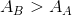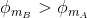# AP Physics C Electricity : Understanding Flux

## Example Questions

### Example Question #1 : Understanding Flux

A uniform magnetic fieldis created in a region in space, as represented by the grey arrows in the diagram below. Four circular loops A, B, C, and D are placed in the magnetic field. The planes of loops A and B are oriented perpendicularly to the direction of the field; the planes of loops C and D are oriented parallel to the field.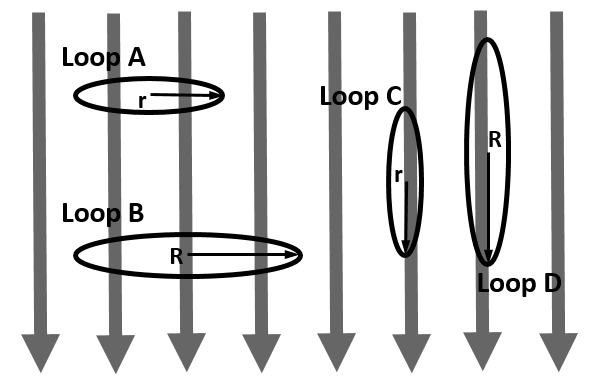Loops A and C are the same size, with radius. Loops B and D are the same size, with radius. Note that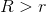.

Which of the statements below is true about the values of magnetic flux through the loops due to the magnetic field?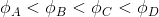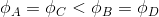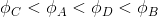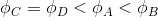Explanation:

Magnetic flux is a measure of the amount of magnetic field passing through a given area and is given by the equation: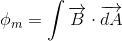The magnetic field is constant for this problem, and the areas are all circular loops. As such, the vector product integral simplifies to: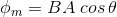The angle theta is the angle between a vector normal to the surface of the areaand the given magnetic field.

For loops C and D, theta is 90 degrees, so the magnetic flux for both of these loops is equal to zero.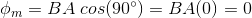For loops A and B, the angle theta is 0 degrees, so the magnetic flux for both of these loops is nonzero and proportional to the area of each loop. Therefore, the flux for loop B is larger than that for loop A because loop B has the larger radius.